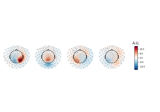# mne.time_frequency.psd_array_welch¶

mne.time_frequency.psd_array_welch(x, sfreq, fmin=0, fmax=inf, n_fft=256, n_overlap=0, n_per_seg=None, n_jobs=1, average='mean', window='hamming', verbose=None)[source]

Compute power spectral density (PSD) using Welch’s method.

Parameters
x`array`, shape=(…, n_times)

The data to compute PSD from.

sfreq`float`

The sampling frequency.

fmin`float`

The lower frequency of interest.

fmax`float`

The upper frequency of interest.

n_fft`int`

The length of FFT used, must be `>= n_per_seg` (default: 256). The segments will be zero-padded if `n_fft > n_per_seg`.

n_overlap`int`

The number of points of overlap between segments. Will be adjusted to be <= n_per_seg. The default value is 0.

n_per_seg

Length of each Welch segment (windowed with a Hamming window). Defaults to None, which sets n_per_seg equal to n_fft.

n_jobs`int`

The number of jobs to run in parallel (default 1). Requires the joblib package.

average

How to average the segments. If `mean` (default), calculate the arithmetic mean. If `median`, calculate the median, corrected for its bias relative to the mean. If `None`, returns the unaggregated segments.

New in version 0.19.0.

window

Windowing function to use. See `scipy.signal.get_window()`.

New in version 0.22.0.

verbose

If not None, override default verbose level (see `mne.verbose()` and Logging documentation for more). If used, it should be passed as a keyword-argument only.

Returns
psds`ndarray`, shape (…, n_freqs) or (…, n_freqs, n_segments)

The power spectral densities. If `average='mean` or `average='median'`, the returned array will have the same shape as the input data plus an additional frequency dimension. If `average=None`, the returned array will have the same shape as the input data plus two additional dimensions corresponding to frequencies and the unaggregated segments, respectively.

freqs`ndarray`, shape (n_freqs,)

The frequencies.

Notes

New in version 0.14.0.

## Examples using `mne.time_frequency.psd_array_welch`¶